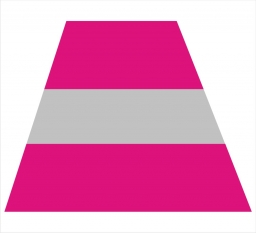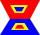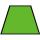# The size

The size of a Trapezium are 3/4×cm, ×cm 2(×+1)cm and 3(×+2)cm long respectively if it's perimeter is 60cm, calculate the length of each side.

a =  3.8519 cm
b =  7.7037 cm
c =  17.4074 cm
d =  29.1111 cm

### Step-by-step explanation:Did you find an error or inaccuracy? Feel free to write us. Thank you!Tips to related online calculators
Do you have a linear equation or system of equations and looking for its solution? Or do you have a quadratic equation?

#### You need to know the following knowledge to solve this word math problem:

We encourage you to watch this tutorial video on this math problem:

## Related math problems and questions:

• Rectangular trapezoidThe ABCD rectangular trapezoid with the AB and CD bases is divided by the diagonal AC into two equilateral rectangular triangles. The length of the diagonal AC is 62cm. Calculate trapezium area in cm square and calculate how many differs perimeters of the
• TrapeziumThe length of the base and the height size of the base of the trapezium is at ratio 5:3:2, the content area of the trapezium is 128 cm2. Calculate the length of the base and the height of the trapezoid.
• Rectangle - sidesWhat is the perimeter of a rectangle with area 266 cm2 if length of the shorter side is 5 cm shorter than the length of the longer side?
• Second sideCalculate the length of the other side of the rectangle if its circumference is 60 cm and one side is 10 cm long.
• Isosceles trapezoidPerimeter of the isosceles trapezoid is 48 cm. One side is two times greater than the second side. Determine the dimensions of the trapezoid.
• Three sidesSide b is 2 cm longer than side c, side a is 9 cm shorter than side b. The triangle circumference is 40 cm. Find the length of sides a, b, c . .. .
• RT leg and perimeterCalculate the length of the sides of a right triangle ABC with hypotenuse c when the length of a leg a= 84 and perimeter of the triangle o = 269.
• BasesThe length of the bases trapezium are in ratio 4:5. Length of midline is 15. How long are the bases of a trapezoid?
• Trapezium diagonalsIt is given trapezium ABCD with bases | AB | = 12 cm, |CD| = 8 cm. Point S is the intersection of the diagonals for which |AS| is 6 cm long. Calculate the length of the full diagonal AC.
• R TrapeziumRectangular trapezium has bases 21 and 10 and area 92 cm2. What is its perimeter?
• Four sides of trapezoidCalculate the area of the ABCD trapezoid with sides a = 65 cm, b = 29 cm, c = 40 cm, d = 36 cm
• Triangle - is RT?Triangle has a circumference of 90 cm. Side b is 1 cm longer than c, and side c is 31 cm longer than side a. Calculate the length of sides and determine whether a triangle is a right triangle.
• IS trapezoidIsosceles trapezoid arm measured 35 cm. Height is 30 cm and middle segment is 65 cm. Determine length of its bases.
• Isosceles trapezoidCalculate the content of an isosceles trapezoid whose bases are at ratio 5:3, the arm is 6cm long and it is 4cm high.
• Isosceles trapezoidCalculate the circumference and the contents of the isosceles trapezoid if you know the size of the bases is 8 and 12 cm and the size of the arms is 5 cm.
• TrapezoidHow long are the trapezoid bases with area 24 cm2 and height 3 cm. One base is 3 times longer than the shorter.
• Right trapezoidThe right trapezoid has bases 3.2 cm and 62 mm long. The shorter leg has a length 0.25 dm. Calculate the lengths of the diagonals and the second leg.